# Number Theory Algorithms

3.6
92 reviews
10K+Everyone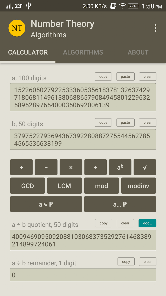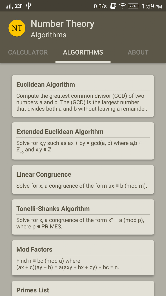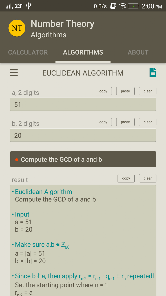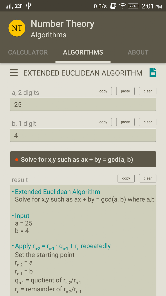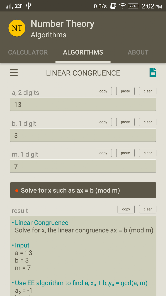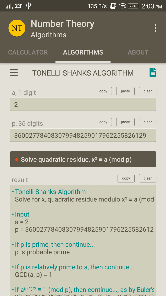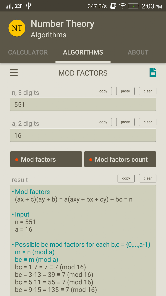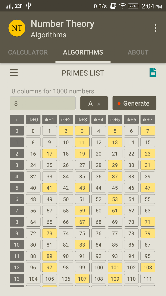Created for personal use this application can handle the following operations:

Calculator:
- add two big integer numbers
- subtract two big integer numbers
- multiply two big integer numbers
- divide two big integer numbers
- power of a big integer number (performance is based on the device)
- root of a big integer number (performance is based on the device)
- GCD of two big integer numbers
- LCM of two big integer numbers
- modulo of two big integer numbers
- modulo inverse of two big integer numbers
- check a big integer if is probable prime
- next probable prime to a big integer

Algorithms:
- Euclidean Algorithm, compute the greatest common divisor (GCD) of two numbers a and b.
- Extended Euclidean Algorithm, solve for x, y such as ax + by = gcd(a, b).
- Linear Congruence, Solve for x, a congruence of the form ax ≡ b (mod m).
- Tonelli-Shanks Algorithm, calculate quadratic residue modulo. Solve for x, a congruence of the form x² ≡ a (mod p) where p is prime.
- Mod-Factors, calculate modular possible factors for a given number and a modulo. Find n ≡ bc (mod a) where (ax + c)(ay + b) = a(axy + bx + cy) + bc = n.
- Primes List, list of primes ordered in columns.
Updated on
Apr 13, 2018

## Data safety

Safety starts with understanding how developers collect and share your data. Data privacy and security practices may vary based on your use, region, and age. The developer provided this information and may update it over time.No data shared with third partiesNo data collected
3.6
92 reviews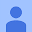February 24, 2019
Overall, a good app for primality and mod calculations. Would be nice to have an option to disable the tactile vibration, which is a bit annoying as it's there on pressing any button.
7 people found this review helpful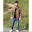Tanmay Tyagi
January 28, 2021
Just what I was looking for. And the copy paste clear commands are so practical and useful. Big thumbs up for the developer!!
3 people found this review helpful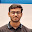SOURASHIS MONDAL
June 13, 2020
Very good app for college students to solve number theoretical problems.... With having a look at the method to solve that
5 people found this review helpful

Calculator: